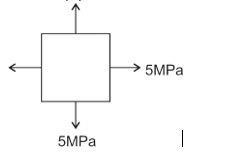Engineering Jobs   »   CIVIL ENGINEERING QUIZ

# DFCCIL’21 CE: Daily Practice Quiz. 20-July-2021

Quiz: Civil Engineering
Exam: DFCCIL-JE
Topic: MISCELLANEOUS

Each question carries 1 mark
Negative marking: 1/4 mark
Time: 8 Minutes

Q1. Shear span is defined as the zone where:
(a) bending moment is zero
(b) shear force is zero
(c) shear force is constant
(d) bending moment is constant

Q2. A stress element is subjected to tensile stress of 5 MPa on both the principal planes. The radius of Mohr Circle corresponding to this element will be
(a) 10 MPa
(b) 7.5 MPa
(c) 5 MPa
(d) Zero

Q3. If the dosage of Alum is 15 mg/l. then the estimated quantity of Alum required (MT) for 10 million liter of water per day will be:
(a) 15
(b) 0.015
(c) 1.5
(d) 0.15

Q4. What is the main disadvantage of Aeration process?
(a) Excessive aeration absorb too much carbon dioxide and water becomes corrosive.
(b) It effectively removes volatile gases which is harmful for water
(c) Excessive aeration absorb too much oxygen and thus water becomes corrosive
(d) It removes oils and decomposes algae and other aquatic product from water

Q5. One stokes is equal to
(a) 1 cm²/s
(b) 1 m²/s
(c) 1 mm²/s
(d) 10 m²/s

Q6. What torque in Nm is required to give 3m³/s of water, a moment of momentum, so that it has a tangential velocity of 3 m/s at a distance of 1.8 m from the axis?
(a) 16200
(b) 157
(c) 2624
(d) 8138

SOLUTION

S1. Ans.(c)
Sol. Shear span is the distance from the point of application of concentrated force to its respective reaction force. throughout shear span the shear force is constant.
“Shear span is defined as the zone where shear force is constant.”

S2. Ans.(d)σx = 5 MPa
σy = 5 MPa
Radius of Moh’r circle = |(σx-σy)/2|
= |(5-5)/2|
= 0
S3. Ans.(d)
Sol. Dosage of alum = 15 mg/l
Total quantity of water treated = 10 million liters
= 10 × 10^6 liter
Quantity of alum required = 15 × 10 × 10^6
= 15 × 10^7 mg
= 150 kg.
= 0.15 MT.

S4. Ans.(c)
Sol. the main disadvantage of the aeration process is that excessive aeration absorbs too much oxygen and thus water becomes corrosive.

S5. Ans.(a)
Sol. We know that (stoke is the unit of kinematic viscosity)
1 stoke = 10^(-4) m²/sec
1 stoke = 10^(-4)×10^4 (cm^2)/sec
1 stoke = 1 cm²/sec

S6. Ans.(a)
Sol. We know that the momentum of water is
F = ρaV²
F = ρQV
F = 1000×3×3
= 9000 N.
Moment of momentum i.e. torque required
τ= f×y
τ =9000×1.8
τ=16200 Nm

Sharing is caring!